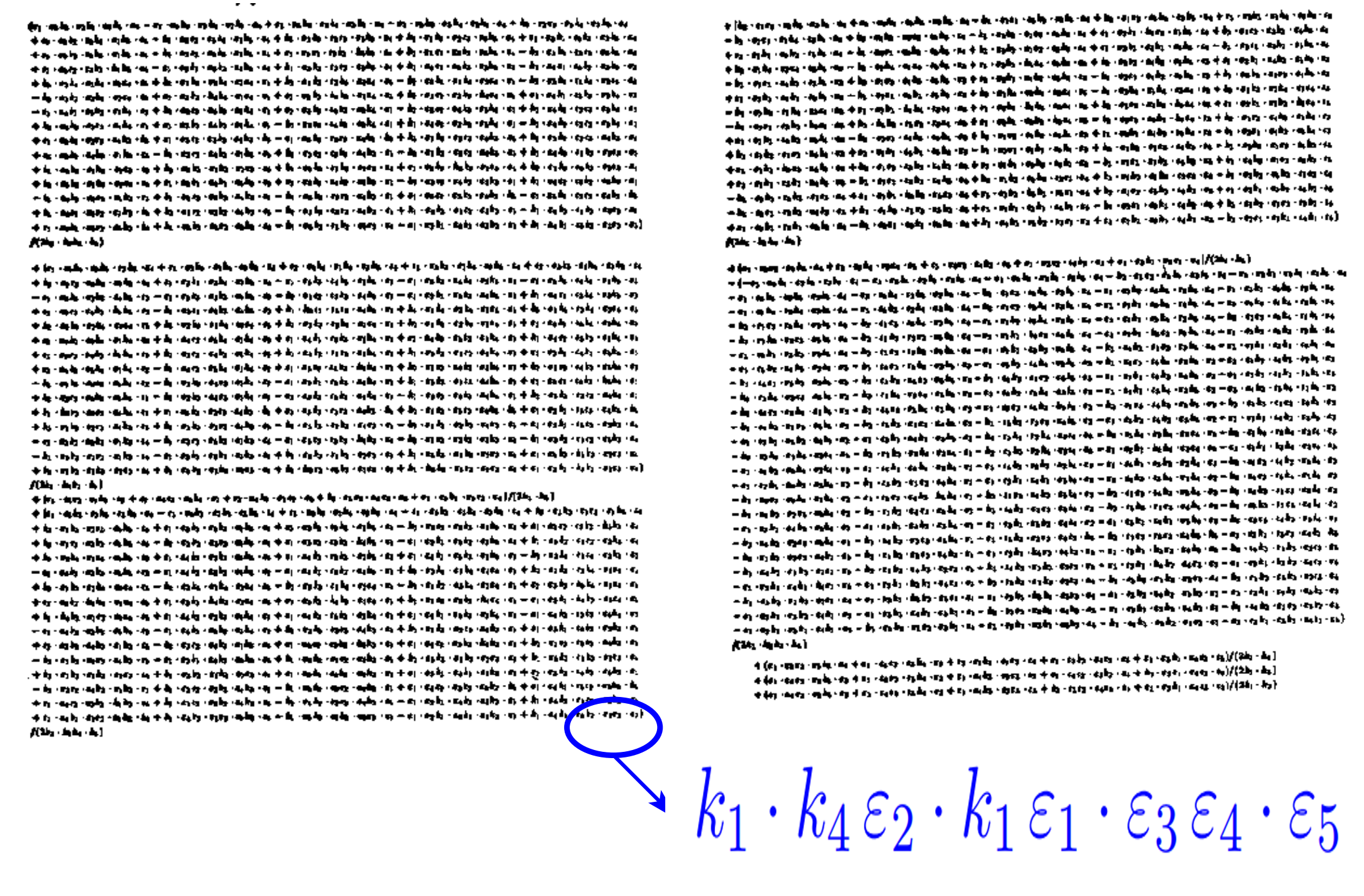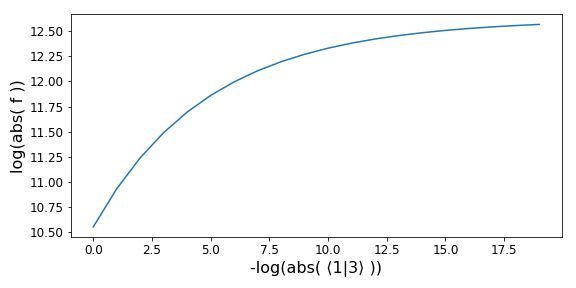Analytical   amplitudes   from   numerical   evaluations

arXiv:1904.04067

Giuseppe De Laurentis

with Daniel Maitre

QCD@LHC 2019

IPPP - Durham UniversityTable  of  Contents

1. Motivation and introduction

2. Singularity structure

3. Ansatz and amplitude reconstruction

4. Some results (Yang-Mills in the Standard Model)

1.1  Motivation

Cross sections at hadron colliders are given by:

$σ_{2 \rightarrow n - 2} = ∑_{a,b} ∫ dx_a dx_b f_{a/h_1}(x_a, μ_F) \, f_{b/h_2}(x_b, μ_F) \;\hat{σ}_{ab→n-2}(μ_F, μ_R)$

$d\hat{σ}_{n}=\frac{1}{2\hat{s}}dΠ_{n-2}\;(2π)^4δ^4\big(∑_{i=1}^n p_i\big)\;|\overline{\mathcal{A}(p_i,μ_F, μ_R)}|^2$

Better predictions require both more loops and higher multiplicity.

 $\mathcal{A}_{mult.}^{loops} \propto g_s^n$ multiplicity 4 5 6 7 loops 0 2 3 4 5 1 4 5 6 7 2 6 7 8 9

Powers of coupling in pure gluon scattering.

We can split dynamics from kinematics with the following identities.

Color ordering at tree level and one loop [1, 2, …]:

$\mathcal{A}^{tree}_{n}({p_i, λ_i, a_i}) = \; g^{n-2} ∑_{σ\in S_n/Z_n} \text{Tr}(T^{a_σ(1)}\dots T^{a_σ(n)}) A^{tree}_n(σ(1^{λ_1}),\dots ,σ(n^{λ_n}))$

$\mathcal{A}^{1-loop}_{n}({p_i, λ_i, a_i}) = \; g^{n} ∑_{σ\in S_n/Z_n} N_{c} \text{Tr}(T^{a_σ(1)}\dots T^{a_σ(n)}) A_{n;1}(σ(1^{λ_1}),\dots ,σ(n^{λ_n}))$ $+ ∑_{c = 2}^{\lfloor n/2 \rfloor + 1}∑_{σ\in S_n/Z_{n;c}} \text{Tr}(T^{a_σ(1)}\dots T^{a_σ(c-1)})\text{Tr}(T^{a_σ( c)}\dots T^{a_σ(n)}) A_{n;c}(σ(1^{λ_1}),\dots ,σ(n^{λ_n}))$

Decomposition in terms of basis integrals [3, 4, 5, …]:

$$A^{1-loop}_{n;1} = \sum_i d_i I^i_{Box} + \sum_i c_i I^i_{Triangle} + \sum_i b_i I^i_{Bubble} + R$$

$A^{tree},\, d_i,\, c_i,\, b_i,$ and $R$ are rational functions of kinematic invariants only.

Brute force calculations are a mess:Results are often much easier [6, 7]:

$A^{tree}(1^{+}_{g}2^{+}_{g}3^{+}_{g}4^{-}_{g}5^{-}_{g}) = \frac{i\,⟨45⟩^{4}}{⟨12⟩⟨23⟩⟨34⟩⟨45⟩⟨51⟩}$

Numerical calculations efficiently bypass algebraic complexity, see:
BlackHat , CutTools , MadGraph , Rocket , Samurai , NGluon , OpenLoops …

But analytical results can still be useful, they can provide:

 $\cdot$ faster computation $\cdot$ better numerical stability $\cdot$ easier to distribute than a program $\cdot$ possible to compile on a GPU $\cdot$ explicit analytical structure $…$

How can we access a (relatively) compact analytical final expression from numerical evaluations?

1.2  Spinor  Helicity

The lowest-laying representations of the Lorentz group are:

(Recall: $\mathfrak{so}(1, 3)_\mathbb{C} \sim \mathfrak{su}(2) \times \mathfrak{su}(2)$)

$\boldsymbol{(j_{-},j_{+})}$ dim. name quantum field kinematic variable
(0,0) 1 scalar $h$ m
(0,12) 2 right-handed Weyl spinor $\chi_{R\,\alpha}$ $\lambda_\alpha$
(12,0) 2 left-handed Weyl spinor $\chi_L^{\,\dot\alpha}$ $\bar{\lambda}^{\dot\alpha}$
(12,12) 4 rank-two spinor/four vector $A^\mu/A^{\dot\alpha\alpha}$ $P^\mu/P^{\dot\alpha\alpha}$
(12,0)$\oplus$(0,12) 4 bispinor (Dirac spinor) $\Psi$ $u, v$

Weyl spinors are sufficient for massless particles:

$\text{det}(P^{\dot\alpha\alpha})=m^2 \rightarrow 0 \quad \Longrightarrow \quad P^{\dot\alpha\alpha} = \bar\lambda^{\dot\alpha}\lambda^\alpha$.

In terms of 4-momentum components we have:

$$\lambda_\alpha=\frac{1}{\sqrt{p^0+p^3}}\begin{pmatrix}p^0+p^3 \\ p^1+ip^2\end{pmatrix} \, , \;\;\; \lambda^\alpha=\epsilon^{\alpha\beta} \lambda_\beta =\frac{1}{\sqrt{p^0+p^3}}\begin{pmatrix}p^1+ip^2 \\ -p^0+p^3\end{pmatrix}$$

$\bar\lambda_{\dot\alpha}=\frac{1}{\sqrt{p^0+p^3}}\begin{pmatrix}p^0+p^3 \\ p^1-ip^2\end{pmatrix} \, , \;\;\; \bar\lambda^{\dot\alpha}=\epsilon^{\dot\alpha\dot\beta}\bar\lambda_{\dot\beta}=\frac{1}{\sqrt{p^0+p^3}}\begin{pmatrix}p^1-ip^2 \\ -p^0+p^3\end{pmatrix}$

The left and right Weyl spinors are related by complex conjugation in the case of real momenta, but are independent for complex momenta.

Some definitions:

$$⟨ij⟩ = λ_iλ_j = (λ_i)^α(λ_j)_α \quad \quad \quad [ij] = \barλ_i\barλ_j = (\barλ_i)_\dotα(\barλ_j)^\dotα$$

$$s_{ij} = ⟨ij⟩[ji]$$

$$⟨i\;|\;(j+k)\;|\;l] = (λ_i)^α (\not P_j + \not P_k )_{α\dotα} \barλ_l^\dotα$$

$$⟨i\;|\;(j+k)\;|\;(l+m)\;|\;n⟩ = (λ_i)^α (\not P_j + \not P_k )_{α \dot α} (\bar{\not P_l} + \bar{\not P_m} )^{\dot α α} (λ_n)_α$$

$$tr_5(ijkl) = tr(\gamma^5 \not P_i \not P_j \not P_k \not P_l) = [i\,|\,j\,|\,k\,|\,l\,|\,i⟩ - ⟨i\,|\,j\,|\,k\,|\,l\,|\,i]$$

2.1  Singular  limits

Singular limits give us information about the poles of the amplitude.

We need a set of possible poles of the amplitudes:

$r_i \in \{ ⟨12⟩, ⟨13⟩, \dots, ⟨1|2+3|4], \dots, s_{123}, \dots \}$,

and let $\, \mathbb{f} \,$ be the function we want to reconstruct.

We want to build phase space points where a single invariant vanishes:

$r_i \rightarrow ε \ll 1, \quad r_{j \neq i} \sim \mathcal{O}(1), \quad \mathbb{f} \rightarrow ε^α \; \Rightarrow \; log(\mathbb{f}) \rightarrow α\cdot log(ε)$

$\Rightarrow$ The slope of the log-log plot gives us the type of singularity, if it exists.

The uniqueness of the singular limit is important to avoid ambiguities, and it can be guaranteed only with complex momenta.

As an example, let us consider the following amplitude:

$\mathbb{f} = A^{tree}(1^{+}_{g}2^{+}_{g}3^{+}_{g}4^{-}_{g}5^{-}_{g}6^{-}_{g})$

 $\lim_{⟨12⟩ \rightarrow \epsilon} \mathbb{f} \propto \epsilon^{-1}$ $\lim_{⟨13⟩ \rightarrow \epsilon} \mathbb{f} \propto \epsilon^0$Note: the invariant on the x-axis gets smaller from left to right.

Studying all the limits yields the least common denominator for $\mathbb{f}$:

$\mathbb{f} = \frac{\mathcal{N_{LCD}}}{\mathcal{D_{LCD}}} = \frac{\mathcal{N_{LCD}}}{⟨12⟩⟨16⟩⟨23⟩⟨34⟩s_{234}s_{345}}$.

The complexity of the numerator depends on two parameters:

1. mass dimension; $\quad \quad \quad$ 2. little group scalings.

In this case $\mathcal{N_{LCD}}$ has mass dimension 10, and phase weights [-1, 0, -1, 1, 0, 1].

The ansatz has 1326 independent terms.

2.2  Doubly  singular  limits

Except for the easiest cases, we should really think about $\mathbb{f}$ as:

$\mathbb{f} = \sum_i \frac{\mathcal{N}_i}{\mathcal{D}_i} = \sum_i \frac{\mathcal{N}_i}{\mathcal{R}_i\mathcal{S}_i}$,

where $\mathcal{R}_i$ are products of subsets of $\mathcal{D_{LCD}}$ (i.e. real poles),
and $\mathcal{S}_i$ are products of factors not in $\mathcal{D_{LCD}}$ (i.e. spurious poles).

This information can be accessed by studying doubly singular limits.

We now want phase space points where two invariants vanish:

$r_i \rightarrow ε \ll 1, \quad r_j \rightarrow ε \ll 1, \quad \mathbb{f} \rightarrow ε^α \; \Rightarrow \; log(\mathbb{f}) \rightarrow α\cdot log(ε)$

In general we cannot guarantee uniqueness anymore,
even with complex momenta.
$\exists \;r_{k \neq i, j} \sim \epsilon$.

Information from taking the doubly singular limits:

⟨1|2⟩ ⟨1|6⟩ [1|6] ⟨2|3⟩ ⟨3|4⟩ [3|4] [4|5] [5|6] s_234 s_345
⟨1|2⟩ 1 1/30 1/3 1/31 1/2 2/12 2/3 2/10 1/2 2/10
⟨1|6⟩ 1/30 1 1/2 1/2 1/10 2/4 2/12 1/3 2/10 2/10
$\dots$
s_234 1/2 2/10 2/10 2/10 2/10 2/10 1/2 2/10 1 1/2
s_345 2/10 2/10 2/10 1/2 2/10 2/10 2/10 1/2 1/2 1

The first number is the slope of the log-log plot in the limit,
the second number is the degeneracy of the phase space in the limit.

Let’s introduce the following notation, which we’ll need later: $\{a, b\}_\epsilon$.
It represents the set of all invariants that vanish when $a$ and $b$ vanish.
For example:
$\{⟨12⟩,\,⟨16⟩\}_\epsilon = \{ ⟨12⟩, ⟨16⟩, ⟨26⟩, s_{345}, ⟨2|1+6|5], \dots \text{25 more} \dots \}$

The slope in the doubly singular limit tells us whether two poles should be in the same denominator and the degeneracy how to separate them.

The following is single line of the table in the previous slide:

Green: forced. Red: forbidden. Blue: optional (at least one). Light blue: optional.
⟨1|2⟩ ⟨1|6⟩ [1|6] ⟨2|3⟩ ⟨3|4⟩ [3|4] [4|5] [5|6] s_234 s_345
s_234 1/2/2 2/10/3 2/10/3 2/10/3 2/10/3 2/10/4 1/2/2 2/10/3 1 1/2/2Diagramatic representation of relation between poles

Let us briefly consider the coefficient of a three mass triangle:$\mathcal{D_{LCD}} = ⟨12⟩⟨34⟩⟨56⟩⟨1|3+4|2]^4⟨3|1+2|4]^4⟨5|1+2|6]^4Δ_{135}^3$

⟨12⟩  ⟨34⟩  ⟨56⟩  ⟨1|3+4|2] ⟨3|1+2|4] ⟨5|1+2|6] Δ_135
⟨1|2⟩ 1 1/2 1/2 2/12 1/2 2/12 4/4 5/6 2/10 2/4
$\dots$
⟨3|1+2|4] 5/6 2/10 4/4 4/4 2/10 5/6 4/2 4 4/2 3.5/4
$\dots$

Do we need square roots of momentum invariants?

All branch cuts should have been taken care of by unitarity cuts.

We should be able to explain this without using square roots.

The culprit is $\Delta$, which first appears in the
three mass triangle momentum parametrisation:

$\Delta_{135} = (K_1 \cdot K_2)^2 - K_1^2 K_2^2$

Solution:
In some limits $\Delta$ behaves like the square of some quantity

$(\Omega_{351})^2 \equiv (2s_{12}s_{56}-(s_{12}+s_{56}-s_{45})s_{123})^2 = 4s_{123}^2\Delta_{135}-4s_{12}s_{56}\langle 4|1+2|3]\langle 3|1+2|4]$

$(\Pi_{351})^2 \equiv (s_{123}-s_{124})^2 = 4\Delta_{135}-4\langle 4|1+2|3]\langle 3|1+2|4]$

3.1  Partial  fraction  decomposition

Going back to our tree level example,
let’s see how we can group together the poles of the amplitude.$\frac{\mathcal{N_1}}{⟨23⟩⟨34⟩⟨2|1+6|5]s_{234}}+\\ \frac{\mathcal{N_2}}{⟨12⟩⟨16⟩⟨2|1+6|5]s_{345}}\phantom{+}$

(Spoiler: $\quad \small \mathcal{N_1} = 1i⟨4|2+3|1]^3, \quad \mathcal{N_2} = -1i⟨6|1+2|3]^3$)

Where does $⟨2|1+6|5]$ come from?

$\{⟨12⟩, \}_{\epsilon} \, \cap \, \{⟨16⟩, s_{234}\}_{\epsilon} \, \cap \, \{⟨23⟩, \}_{\epsilon} \, \cap \, \{, s_{234}\}_{\epsilon} \, \dots$$\frac{\mathcal{N_1}}{⟨16⟩⟨1|2+3|4]⟨5|1+6|2]s_{234}}+\\$ $\frac{\mathcal{N_2}}{⟨34⟩⟨3|1+2|6]⟨5|1+6|2]s_{345}}+\\$ $\frac{\mathcal{N_3}}{⟨12⟩⟨23⟩⟨1|2+3|4]⟨3|1+2|6]}$

(Spoiler: $\quad \small \mathcal{N_1} = 1i^3⟨56⟩^3, \quad \mathcal{N_2} = -1i^3⟨45⟩^3, \quad \mathcal{N_3} = 1is_{123}^3$)

$⟨1|2+3|4] = \{⟨12⟩, \}_{\epsilon} \, \cap \, \{⟨23⟩, s_{234}\}_{\epsilon} \, \cap \, \{⟨16⟩, \}_{\epsilon} \, \cap \, \dots$ $⟨3|1+2|6] = \{⟨23⟩, \}_{\epsilon} \, \cap \, \{⟨12⟩, s_{345}\}_{\epsilon} \, \cap \, \{⟨34⟩, \}_{\epsilon} \, \cap \, \dots$

Both of these partial fractions correspond to some BCFW shift,
but we are not limited to these representations.$\frac{\mathcal{N_1}}{⟨23⟩⟨34⟩s_{234}s_{345}}+\\$ $\frac{\mathcal{N_2}}{⟨12⟩⟨16⟩s_{234}s_{345}}+\\$ $\frac{\mathcal{N_3}}{⟨12⟩⟨23⟩s_{234}s_{345}}$

($\small \mathcal{N_1} = 1i⟨45⟩⟨4|2+3|1]^2, \, \mathcal{N_2} = 1i⟨56⟩⟨6|1+2|3]^2, \, \mathcal{N_3} = 1i⟨4|2+3|1]⟨6|1+2|3]s_{123}$)

We now have no spurious poles, but $s_{234}$ and $s_{345}$ appear in the same denominator, although the doubly singular limit suggests they shouldn’t.

This means that in the limit of $\{s_{234}, s_{345}\} \rightarrow \epsilon$ the individual terms will behave like $\epsilon^{-2}$ but the sum as $\epsilon^{-1}$.

Different representations can be exploited to ensure numerical stability.

3.2  Fitting  of  ansatz

Let’s consider the first representation:

$\mathbb{f}=$ $\frac{\mathcal{N_1}}{⟨23⟩⟨34⟩⟨2|1+6|5]s_{234}}+$ $\frac{\mathcal{N_2}}{⟨12⟩⟨16⟩⟨2|1+6|5]s_{345}}$

$\mathcal{N_1}$ has mass dimension: 6, and phase weights: [-3, 0, 0, 3, 0, 0].
We generate all possible products of spinor products compatible with this infomation. The ansatz has size 10:

$[⟨24⟩⟨24⟩⟨24⟩,$ $⟨24⟩⟨24⟩⟨34⟩,$ $⟨24⟩⟨24⟩⟨45⟩,$ $⟨24⟩⟨34⟩⟨34⟩,$ $⟨24⟩⟨34⟩⟨45⟩,$ $⟨24⟩⟨45⟩⟨45⟩,$ $⟨34⟩⟨34⟩⟨34⟩,$ $⟨34⟩⟨34⟩⟨45⟩,$ $⟨34⟩⟨45⟩⟨45⟩,$ $⟨45⟩⟨45⟩⟨45⟩]$,

much smaller than the one for $\mathcal{N_{LCD}}$
which had more than 1000 terms!

We want to isolate the first term in

$\mathbb{f}=$ $\frac{\mathcal{N_1}}{⟨23⟩⟨34⟩⟨2|1+6|5]s_{234}}+$ $\frac{\mathcal{N_2}}{⟨12⟩⟨16⟩⟨2|1+6|5]s_{345}}$

this can be done by generating phase space points
in the limit of, say, $s_{234} \rightarrow \epsilon$.

Then we can reconstruct the numerical coefficient of the ansatz entries by Gaussian elimination.

$$M_{ij}c_j = \mathbb{f}(P_i) \quad \text{i.e.} \quad \begin{pmatrix} \leftarrow ansatz(P_1) \rightarrow \\ \leftarrow ansatz(P_2) \rightarrow \\ \dots \end{pmatrix} \cdot \begin{pmatrix} c_1 \\ c_2 \\ \dots \end{pmatrix} = \begin{pmatrix} \mathbb{f}(P_1) \\ \mathbb{f}(P_2) \\ \dots \end{pmatrix}$$

We get:

$⟨24⟩⟨24⟩⟨24⟩: 1i$, $⟨24⟩⟨24⟩⟨34⟩: 3i$,
$⟨24⟩⟨34⟩⟨34⟩: 3i$, $⟨34⟩⟨34⟩⟨34⟩: 1i$

How big is the ansatz?

Easiest to count at constant null phase weights;
the size of the ansatz is a function of:

1. its mass dimension ($d$) $\quad\quad$ 2. multiplicity of phase space ($m$).

If we allow only for a polynomial in the numerator, then:

$|s_{ij}| = \frac{m(m-3)}{2}$ $\quad\quad$ $|tr_5| = {m-1 \choose 4}$

$\left(\mkern -9mu \begin{pmatrix}\, |s_{ij}| \, \\ \, d/2 \, \end{pmatrix} \mkern -9mu \right) \leq$ ansatz size $\leq \left(\mkern -9mu \begin{pmatrix} \, |s_{ij}| \, \\ \, d/2 \, \end{pmatrix} \mkern -9mu \right) + |tr_5| \left(\mkern -9mu \begin{pmatrix} \, |s_{ij}| \, \\ \, (d-4)/2 \, \end{pmatrix} \mkern -9mu \right)$

The upper bound is saturated for $(\forall m \wedge d \leq 4)$ and for $(\forall d \wedge m \leq 5)$.
Otherwise it is an overcounting due to Schouten identity for 4-momenta: $tr_5(2345)1_\mu - tr_5(1345)2_\mu + tr_5(1245)3_\mu - tr_5(1235)4_\mu + tr_5(1234)5_\mu = 0$

4.1  Application  and  Results

As an application we obtained analytical expressions for all parts of the six-gluon amplitude with a gluon in the loop.

All independent coefficients are available as anchillary files at: arXiv:1904.04067.

These expression were already present in the litterature, but with different structures. We did not use any information from those papers for the reconstruction.

For a summary of the litterature see Ref.15.

For the three mass triangle mentioned in an earlier section, which was previously obtained in , the poles are:

$\mathcal{D_{LCD}} = ⟨12⟩⟨34⟩⟨56⟩⟨1|3+4|2]^4⟨3|1+2|4]^4⟨5|1+2|6]^4Δ_{135}^3$Symmetries: $123456$ $\overline{216543}$ $345612$ $\overline{432165}$ $561234$ $\overline{654321}$

We obtain an explicitly rational expression, like for $\mathcal{N}=1$ SUSY in .

Another interesting piece is the rational part:

$R^{1-loop}_6(1^{+}_{g}2^{-}_{g}3^{+}_{g}4^{-}_{g}5^{+}_{g}6^{-}_{g})$

$\mathcal{D_{LCD}} =$ $⟨12⟩⟨13⟩^2⟨15⟩^2⟨16⟩⟨23⟩^2^2⟨34⟩⟨35⟩^2⟨45⟩^2⟨56⟩$ $⟨1|2+3|1]^2⟨1|5+6|1]^2⟨1|3+4|2]^2⟨1|2+3|6]^2⟨2|1+6|2]^2⟨2|3+4|2]^2$ $⟨3|1+6|2]^2⟨3|1+2|3]^2⟨3|4+5|3]^2⟨3|1+2|4]^2⟨4|2+3|4]^2⟨4|5+6|4]^2$ $⟨5|1+6|4]^2⟨5|1+6|5]^2⟨5|3+4|5]^2⟨5|1+2|6]^2⟨6|1+2|6]^2⟨6|4+5|6]^2$ $s_{123}s_{234}s_{345}Δ_{135}^2Δ_{624}^2$

The mass dimension is now 116,
which would imply an ansatz with size roughly $10^{10}$.

An analytical expression for this component was previously obtained in  from Feynman diagrams.

The pole structure is a bit of a mess:4.2  Conclusions

The aim of this talk was to show that it is possible to reconstruct an analytical expression from numerical evaluations only.

We discussed a general framework to perform these operations,
and strategies to tame the complexity of the problem.

The application given as an example is representative of the complexity of high multiplicity processes and demonstrates the viability of this approach.

Outlook:
We have now almost finished reconstructing the six-gluon one-loop amplitude with a fermion loop, and we are considering applications to processes with external quarks and photons, as well as to coefficients of two-loop integrals.

Thank you very much!

Questions?